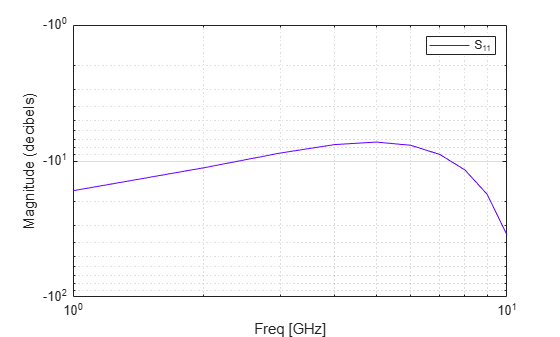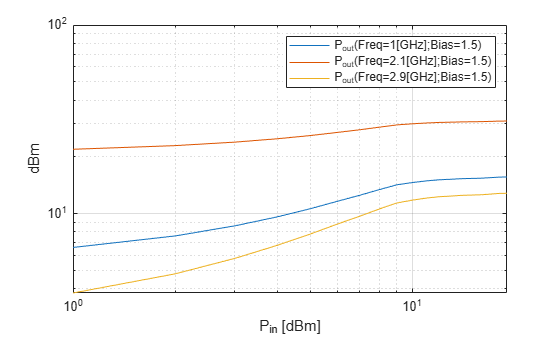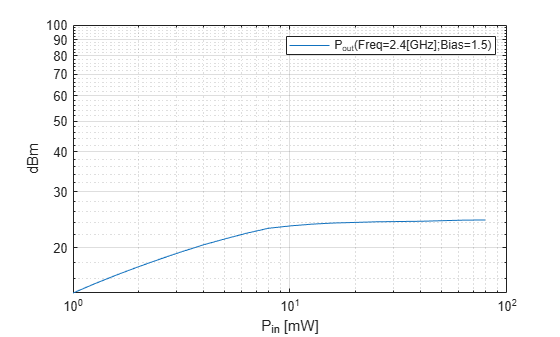# loglog

Plot specified circuit object parameters using log-log scale

## Syntax

``loglog(h,circuitPara)``
``loglog(h,circuitPara,dataFormat)``
``loglog(___,xAxisPara,xAxisFmt)``
``loglog(___,opCon,opVal)``
``loglog(___,Name,Value)``
``lineseries = loglog(___)``

## Description

example

````loglog(h,circuitPara)` plots the circuit parameter `circuitPara` from the RFCKT or RF data object `h` using a log-log scale. You can specify multiple circuit parameters in this syntax. NoteFor all circuit objects except those that contain data from a data file, you must perform a frequency domain analysis with the `analyze` method before calling `loglog`. ```
````loglog(h,circuitPara,dataFormat)` plots the data of the RFCKT or RF data object using a log-log scale with the specified data format. ```

example

````loglog(___,xAxisPara,xAxisFmt)` plots the circuit parameters `circuitPara` using a log-log scale along with the variables `xAxisPara` and their corresponding format `xAxisFmt`. Specify `xAxisPara` and `xAxisFmt` arguments after any of the input argument combinations in the previous syntaxes.```
````loglog(___,opCon,opVal)` plots the circuit parameters using a log-log scale with operating conditions `opCon` and operating values `opVal` for the circuit object `h`. Derive operating conditions for the RFCKT or RF data object `h` using the `getop(h)` command```

example

````loglog(___,Name,Value)` plots the data of a RFCKT or RF data object with name-value arguments. ```
````lineseries = loglog(___)` returns the line series property object `lineseries`. This output is the same as the output returned by the MATLAB® `loglog` function.```

## Examples

collapse all

Create and analyze a two-wire transmission line RFCKT object.

```h = rfckt.twowire('Radius',7.5e-4); freq = linspace(1e9,10e9,10); analyze(h,freq);```

Plot S11 using the log-log scale.

`lineseries = loglog(h,'S11');`

Change the color of the S11 data.

`lineseries(1).Color = [0.4 0 1];`Create an amplifier object from the specified P2D file type.

`h = read(rfckt.amplifier,'default.p2d');`

Plot the output power of the amplifier.

`loglog(h,'Pout')`Plot the output power of the amplifier and set the format for `Pin` in milliwatts.

`loglog(h,'Pout','Pin','mW')`Plot the output power of the amplifier at `2.4` GHz.

`loglog(h,'Pout','Pin','mW','bias',1.5,'Freq',2.4e9)`## Input Arguments

collapse all

RFCKT or RF data object, specified as a `rfckt` or `rfdata` object.

For complete list of RFCKT and RF data objects, see RF Circuit Objects and RF Data Objects.

Format of the data to be plotted, specified as character vector or string scalar. Type `listformat(h,circuitPara)` command to see the available formats for a specified parameter.

Example: `lineseries = semilogy(h,'Pout','dBm')`

Valid RFCKT or data object parameter, specified as a character vector or string scalar.

Use `listparam(h)` for a list of valid parameters for the circuit or data object `h`.

X-axis variable to plot with the circuit parameters, `circuitPara`, specified as a character vector or string scalar.

This table shows the commonly used `circuitPara` and their corresponding `xAxisPara` values. The function uses the default values listed in the table if you do not specify `xAxisPara` .

`circuitPara` Value`xAxisPara` Value
`Pout`, `Phase`, `LS11`, `LS12`, `LS21`, `LS22``Pin` (default), `Freq`
`S11`, `S12`, `S21`, `S22`, `NF`, `IIP3`, `OIP3`, `GroupDelay`, `VSWRIn`, `VSWROut`, `GammaIn`, `GammaOut`, `FMIN`, `GammaOPT`, `RN`, `TF1`, `TF2`, `Gt`, `Ga`, `Gp`, `Gmag`, `Gmsg`, `GammaMS`, `GammaML`, `K`, `Delta`, `Mu`, `MuPrime``Freq`
`AM/AM`, `AM/PM``AM`

`xAxisPara` format, specified as a character vector or string scalar. You do not need to specify `xAxisFmt` when `xAxisPara` is an operating condition.

This table shows the commonly used `xAxisPara` and their corresponding `xAxisFmt`. The function uses the default values listed in the table if you do not specify `xAxisFmt` .

`xAxisPara` Value`xAxisFmt` Value
`Pin``dBm` (default), `mW`, `W`, `dBW`
`Freq`

`THz`, `GHz`, `MHz`, `KHz`, `Hz`

By default, `xAxisFmt` is chosen to provide the best scaling for the given `xAxisPara` values.

`AM``Magnitude (decibels)` (default), ```Magnitude (linear)```

Example: `loglog(h,'Pout','Pin','mW')` plots data using a log-log scale for circuit object, `h`, with `xAxisPara` set to `'Pin'` and `xAxisFmt` set to `'mW'`.

Operating conditions derived from a P2D or S2D file, specified as a string scalar or a character vector.

For some circuit parameters, you can specify a set of frequency or input power values at which the function plots the specified parameter.

For example:

• When plotting large-signal S-parameters as a function of input power, you can specify frequency points of interest using `opCon` and `opVal` arguments.

• When plotting large-signal S-parameters as a function of frequency, you can specify input power levels of interest using `opCon` and `opVal` arguments.

• When plotting parameters as a function of an operating condition, you can specify both frequency and input power values using `opCon` and `opVal` arguments.

Enter the `getop(h)` command at the command line to get the operating conditions for the RF circuit object `h`.

Value of the operating conditions specified using the `opCon` argument, specified as a scalar.

Example: `loglog(h,'Pout','Pin','mW','bias',1.5)` plots the data using a log-log scale for circuit object, `h`, with `opCon` set to `'bias'` and `value` set to `1.5`.

### Name-Value Pair Arguments

Specify optional comma-separated pairs of `Name,Value` arguments. `Name` is the argument name and `Value` is the corresponding value. `Name` must appear inside quotes. You can specify several name and value pair arguments in any order as `Name1,Value1,...,NameN,ValueN`.

Example: `loglog(h,'Pout','Pin','mW','bias',1.5,'Freq',2.4)`

Frequency value used to plot the data using a log-log scale, specified as the comma-separated pair consisting of `'Freq'` and a positive scalar in Hz.

Input power level used to plot the data using a log-log scale, specified as the comma-separated pair consisting of `'Pin'` and a scalar in dBm.

## Output Arguments

collapse all

Lineseries object, returned as a column vector of object handles.

## Tips

• Use the Property Editor ( `propertyeditor` ) or the MATLAB `set` function to change Line Properties. The reference pages for MATLAB functions such as `figure`, `axes`, and `text` also list available properties and provide links to more complete property descriptions.

Note

Use the MATLAB `loglog` function to create a log-log scale plot of parameters that are specified as vector data and are not part of a circuit ( `rfckt` ) object or data ( `rfdata` ) object.

• If `h` has multiple operating conditions, such as from a `.p2d` or `.s2d` file, the `loglog` function operates as follows:

• If you do not specify any operating conditions as arguments to the `loglog` method, then the method plots the parameter values based on the currently selected operating condition.

• If you specify one or more operating conditions, the `loglog` method plots the parameter values based on those operating conditions.

• When you use an operating condition for the `xparameter` input argument, the method plots the parameters for all operating condition values.